Function Repository Resource:

Get a numerically sorted list of abscissa-weight pairs for various modifications of Gaussian quadrature

Contributed by: Jan Mangaldan
 ResourceFunction["ModifiedGaussianQuadratureWeights"][type,n] gives a list of the n pairs {xi,wi} of the n-point modified Gaussian formula for quadrature on the interval -1 to 1, where wi is the weight of the abscissa xi. ResourceFunction["ModifiedGaussianQuadratureWeights"][type,n,{a,b}] rescales the Gaussian formula for the interval a to b. ResourceFunction["ModifiedGaussianQuadratureWeights"][type,n,{a,b},prec] uses the working precision prec.

## Details

Modified Gaussian quadrature approximates the value of an integral as a linear combination of values of the integrand evaluated at optimal abscissas xi: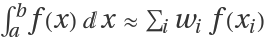.
Modified Gaussian quadrature formulae are obtained as appropriate modifications of the usual Gauss-Legendre quadrature formula to incorporate endpoint or error information.
The following modification types are supported:
 "AntiGaussian" Laurie's anti-Gaussian quadrature formula "Lobatto" Gauss–Lobatto quadrature with both endpoints fixed,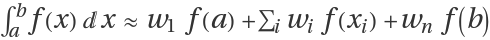"RadauLeft" Gauss–Radau quadrature with left endpoint fixed,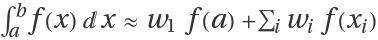"RadauRight" Gauss–Radau quadrature with right endpoint fixed,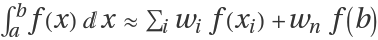The precision argument acts similarly to the WorkingPrecision option used in many Wolfram Language numeric functions; it is not at all like the PrecisionGoal option. If the n argument is too small to allow for a high-precision result, extra precision in a result will not be meaningful.

## Examples

### Basic Examples (3)

The abscissas and weights for a 10-point Lobatto quadrature rule:

 In:=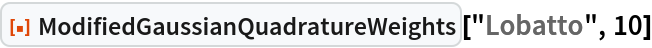Out=Lobatto quadrature rule over the interval 0 to 1:

 In:=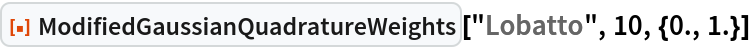Out=Use the specified precision:

 In:=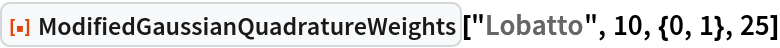Out=### Scope (2)

The abscissas and weights for a 10-point anti-Gaussian rule:

 In:=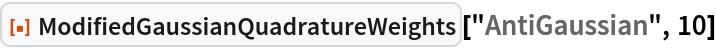Out=The "RadauLeft" and "RadauRight" rules are basically reflections of each other over the integration interval:

 In:=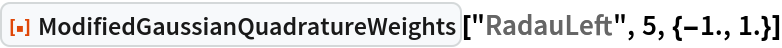Out=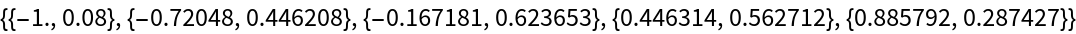In:=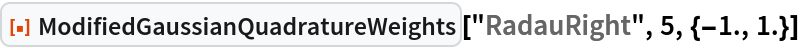Out=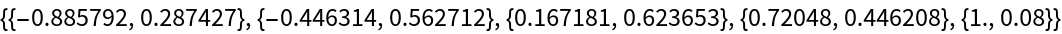### Applications (3)

Use the modified Gaussian quadrature to approximate the area under a curve:

 In:=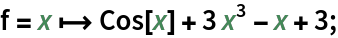Compare the results of the various modifications with classical Gaussian quadrature for a fixed n:

 In:=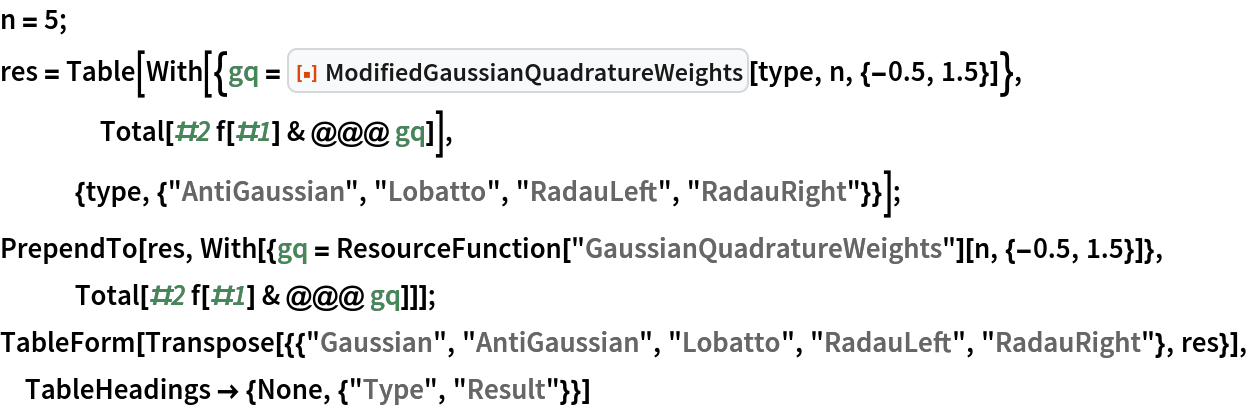Out=Compare with the result of NIntegrate by computing the absolute error:

 In:=Out=## Version History

• 1.0.0 – 03 May 2021